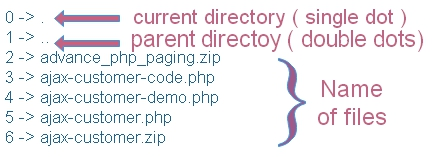# Listing of all types of file extensions with total numbers present inside a directory.

This script will display total number of files with different file extensions present inside a directory.

To get all the files including directory and dots ( representing current director and parent directory ) we can use scandir() function.
``````\$path = '../../php_tutorial/'; // change the path
\$ar=scandir(\$path); // Array with all file and directory names``````
From this we will remove the current directory and parent directory dots ( single and double dots ) by using array_diff()
``\$ar=array_diff(\$ar,array('.','..'));// remove dots``We will create a function `my_pathinfo()` to get the name of file extensions of the elements of the array. We will use array_map() function to apply our function `my_pathinfo()` on all the elements ( output of scandir() ) of the array.
``````\$extensions=array_map('my_pathinfo',\$ar); // Array with all file extensions
// function to get file extensions //
function my_pathinfo(\$str){
return (pathinfo(\$str, PATHINFO_EXTENSION)); // return the file extension
}``````
We will use array_count_values() to create an array with extensions used and frequency of their occurrence.
``\$result=array_count_values(\$extensions);``
We will display all the elements of the array
``````while (list (\$key, \$val) = each (\$result)) {
echo "\$key ( \$val ) <br>";
}``````
The full code is here. Change the \$path to match your directory path.
``````<?Php
\$path = '../../php_tutorial/'; // change the path
\$ar=scandir(\$path); // Array with all file and directory names
\$ar=array_diff(\$ar,array('.','..'));// remove dots

// Each element is executed through callback function //
\$extensions=array_map('my_pathinfo',\$ar); // Array with all file extensions
// function to get file extensions //
function my_pathinfo(\$str){
return (pathinfo(\$str, PATHINFO_EXTENSION)); // return the file extension
}
//Count the frequency of occurrence of elements //
\$result=array_count_values(\$extensions);
while (list (\$key, \$val) = each (\$result)) {
echo "\$key ( \$val ) <br>";
}
?>``````
Here is the output
``````zip ( 5 )
php ( 61 )
txt ( 6 )
htm ( 3 )
js ( 3 )
css ( 3 )
xml ( 3 )
( 7 )
csv ( 2 )
jpg ( 2 ) ``````
There are 7 directories present so there is a listing of number 7 without any file extension

Subscribe to our YouTube Channel here

## Subscribe

* indicates required
Subscribe to plus2netplus2net.com